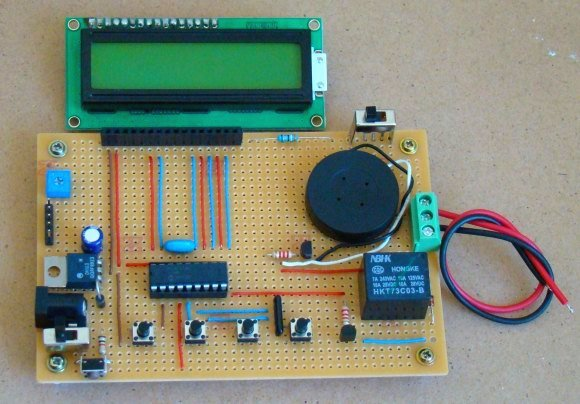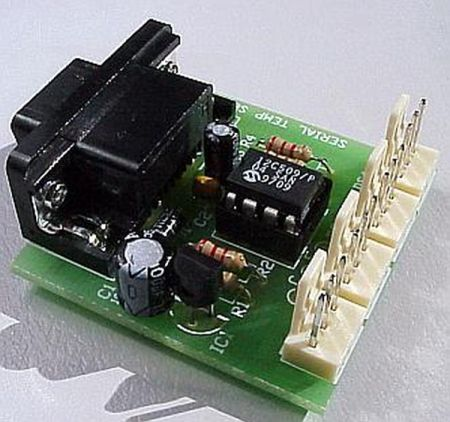# Electronic Code locking system using PIC 16F877 Mircocontroller

Electronic code locking system is extremely useful in protecting our precious possessions and can be installed anywhere with bit of engineering in it. We are widely familiar with the Password based e-locks and might have installed in our house. But we are going for Electronic locks made by any company when you can make one by your own.

This project demonstrates you how to make a PIC microcontroller based simple digital lock and also explains the programming behind it.

## WHAT YOU NEED:

1. PIC 16F877 Microcontroller
2. 4×3 Keypad – Key Input
3. 16×2 LCD – Status Indicator
4. 5V Relay – Activator

Additionally you need a POT which is used to adjust the contrast of the LCD, A transistor to drive the relay since the current obtained from a Pin of Microcontroller is very less. A diode to prevent the reverse flow of current which might damage the controller.

##DESIGN OF ELECTRONIC CODE LOCKING SYSTEM:

The design of the above Embedded Project is pretty straight forward, you need to interface a LCD to PORT B and Keypad to the PORT D of the Controller. If you are not familiar with it then i suggest you to go through this Interfacing LCD and Keypad with PIC Microcontroller. Now lets see how the system is designed to work as a Locker.

• “*” Key – This key is meant to initialize the system, when the system is turned ON the Controller will scan only this key and pressing this key will enable you to enter the password for your locker.
• “#” Key – When you are done with your locker , you should press this key which will turn off the system and turning off the relay as well.
READ  PIC16F877 based digital clock using LCD display (Code+Proteus simulation)

The remaining keys are meant to feed the character values to the Microcontroller and in turn the Microcontroller will analyze the characters. Based on the Pre defined password in the Controller it will compare the input with it. Thereby it will recognize the correct or incorrect password input.

## CODE:

The below code was built using CCS compiler for the PIC Microcontroller
1. #include<16F877.h>
2. #include<stdio.h>
3. #bit led=0x05.0
4. #bit TRIS_led=0x85.0
5. #byte lcd=0x06
6. #byte TRIS_lcd=0x86
7. #bit rs=0x07.0
8. #bit TRIS_rs=0x87.0
9. #bit en=0x07.1
10. #bit TRIS_en=0x87.1
11. #bit relay=0x07.2
12. #bit TRIS_relay=0x87.2
13. #bit C1=0x08.0
14. #bit C2=0x08.1
15. #bit C3=0x08.2
16. #bit R1=0x08.3
17. #bit R2=0x08.4
18. #bit R3=0x08.5
19. #bit R4=0x08.6
20. #bit TRIS_C1=0x88.0
21. #bit TRIS_C2=0x88.1
22. #bit TRIS_C3=0x88.2
23. #bit TRIS_R1=0x88.3
24. #bit TRIS_R2=0x88.4
25. #bit TRIS_R3=0x88.5
26. #bit TRIS_R4=0x88.6
27. void display(unsigned char a,int b); //LCD subroutine
28. char keypad(); //Keypad Subroutine
29. void check(); //Password check routine
30. char password={“7196”}; //Predefined password
31. char pswd;
32. unsigned char open_msg=“Enter Password”;
33. unsigned char welcome_msg=“Welcome”;
34. unsigned char close_msg=“Wrong Password”;
35. char c;
36. int flag,i,count,j;
37. void main()
38. {
39. TRIS_lcd=TRIS_en=TRIS_rs=TRIS_led=TRIS_relay=; //Directions set
40. TRIS_R1=TRIS_R2=TRIS_R3=TRIS_R4=count=;
41. TRIS_C1=TRIS_C2=TRIS_C3=1;
42. while(TRUE)
43. {
44. c=keypad();
45. {
46. if(c==‘*’) //Initialize condition
47. {
48. flag=1; //Flag set to scan other keys
49. count=;
50. display(0x01,);
51. display(0x38,);
52. display(0x0f,);
53. display(0x80,);
54. for(i=;i<=13;i++)
55. {
56. display(open_msg[i],1);
57. }
58. display(0xc0,);
59. }
60. else if(c==‘#’) //Turning off condition
61. {
62. count=;
63. relay=;
64. display(0x01,);
65. display(0x0c,);
66. }
67. else
68. {
69. display(‘*’,1);
70. pswd[count]=c; //Storing input in new arrays
71. count=count+1;
72. check();
73. }
74. }
75. }
76. }
77. void display(unsigned char a,int b)
78. {
79. lcd=a;
80. rs=b;
81. en=1;
82. delay_ms(10);
83. en=;
84. delay_ms(10);
85. }
86. char keypad()
87. {
88. if(flag==) //Waiting for Initialization
89. {
90. while(TRUE)
91. {
92. R4=1;
93. R1=R2=R3=;
94. if(C1==1)
95. {
96. while(C1==1);
97. count=;
98. return ‘*’;
99. }
100. if(C3==1)
101. {
102. while(C3==1);
103. count=;
104. return ‘#’;
105. }
106. }
107. }
108. else if(flag==1)
109. {
110. while(TRUE) //Keypad scan
111. {
112. R1=1;
113. R2=R3=R4=;
114. if(C1==1)
115. {
116. while(C1==1);
117. return ‘1’;
118. }
119. if(C2==1)
120. {
121. while(C2==1);
122. return ‘2’;
123. }
124. if(C3==1)
125. {
126. while(C3==1);
127. return ‘3’;
128. }
129. R2=1;
130. R1=R3=R4=;
131. if(C1==1)
132. {
133. while(C1==1);
134. return ‘4’;
135. }
136. if(C2==1)
137. {
138. while(C2==1);
139. return ‘5’;
140. }
141. if(C3==1)
142. {
143. while(C3==1);
144. return ‘6’;
145. }
146. R3=1;
147. R1=R2=R4=;
148. if(C1==1)
149. {
150. while(C1==1);
151. return ‘7’;
152. }
153. if(C2==1)
154. {
155. while(C2==1);
156. return ‘8’;
157. }
158. if(C3==1)
159. {
160. while(C3==1);
161. return ‘9’;
162. }
163. R4=1;
164. R1=R2=R3=;
165. if(C1==1)
166. {
167. while(C1==1);
1. }
2. if(C2==1)
3. {
4. while(C2==1);
5. return ‘0’;
6. }
7. if(C3==1)
8. {
9. while(C3==1);
10. return ‘#’;
11. }
12. }
13. }
14. }
15. void check()
16. {
17. if(count>3) //Input exceeds count 3 will execute comparison
18. {
19. flag=count=;
20. j=strcmp(pswd,password); //Comparison of input and Predefined pswd
21. if(j==)
22. {
23. relay=1; //Turning relay on
24. display(0x01,);
25. display(0x80,);
26. for(i=;i<=6;i++)
27. {display(welcome_msg[i],1);}
28. }
29. else
30. {
31. relay=;
32. display(0x01,);
33. display(0x80,);
34. for(i=;i<=13;i++)
35. {display(close_msg[i],1);}

READ  Adapter for small size PIC/AVR chip programming

For more detail:  Electronic Code locking system using PIC 16F877 Mircocontroller

#### Current Project / Post can also be found using:

• digital code lock using pic16f877a
• #bit TRIS_relay=0x87 2
• electronick lock system using pic and ccd
• electronic lock using pic16f877a

© 2012 Powered By PIC-Microcontroller.com

###### Read previous post:##### Quozl’s Temperature Sensor Project using PIC12C509

Introduction Just a handful of components builds an 8-pin microcontroller based circuit for temperature logging via a serial port; small,...

Close